#### The van der Corput sequence

A beautiful yet underutilized sequence was published in 1935 by van der Corput, a Dutch mathematician . It exhibits many ideal qualities for applications. At the same time, it is based on a simple idea. Unfortunately, it is only defined for the unit interval. The quest to extend many of its qualities to higher dimensional spaces motivates the formal quality measures and sampling techniques in the remainder of this section.

To explain the van der Corput sequence, let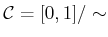, in which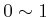, which can be interpreted as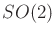. Suppose that we want to place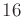samples in. An ideal choice is the set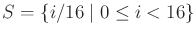, which evenly spaces the points at intervals of length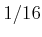. This means that no point inis further than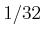from the nearest sample. What if we want to make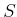into a sequence? What is the best ordering? What if we are not even sure thatpoints are sufficient? Maybeis too few or even too many.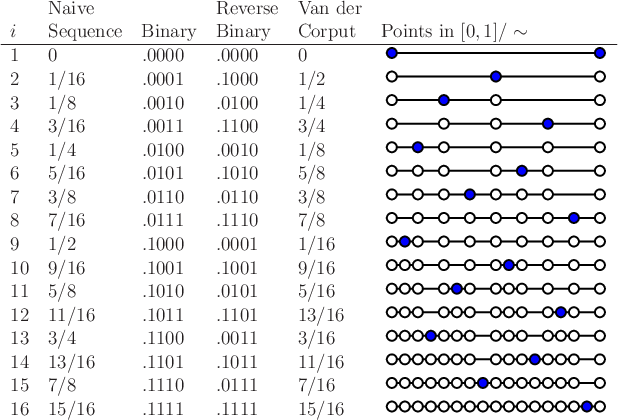The first two columns of Figure 5.2 show a naive attempt at makinginto a sequence by sorting the points by increasing value. The problem is that after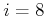, half ofhas been neglected. It would be preferable to have a nice covering offor any. Van der Corput's clever idea was to reverse the order of the bits, when the sequence is represented with binary decimals. In the original sequence, the most significant bit toggles only once, whereas the least significant bit toggles in every step. By reversing the bits, the most significant bit toggles in every step, which means that the sequence alternates between the lower and upper halves of. The third and fourth columns of Figure 5.2 show the original and reversed-order binary representations. The resulting sequence dances around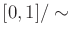in a nice way, as shown in the last two columns of Figure 5.2. Let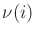denote theth point of the van der Corput sequence.

In contrast to the naive sequence, eachlies far away from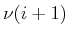. Furthermore, the firstpoints of the sequence, for any, provide reasonably uniform coverage of. Whenis a power of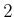, the points are perfectly spaced. For other, the coverage is still good in the sense that the number of points that appear in any interval of length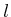is roughly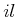. For example, when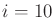, every interval of length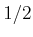contains roughly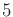points.

The length,, of the naive sequence is actually not important. If instead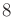is used, the same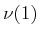,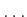,are obtained. Observe in the reverse binary column of Figure 5.2 that this amounts to removing the last zero from each binary decimal representation, which does not alter their values. If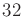is used for the naive sequence, then the same,,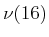are obtained, and the sequence continues nicely fromto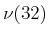. To obtain the van der Corput sequence fromto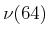, six-bit sequences are reversed (corresponding to the case in which the naive sequence has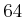points). The process repeats to produce an infinite sequence that does not require a fixed number of points to be specified a priori. In addition to the nice uniformity properties for every, the infinite van der Corput sequence is also dense in. This implies that every open subset must contain at least one sample.

You have now seen ways to generate nice samples in a unit interval both randomly and deterministically. Sections 5.2.2-5.2.4 explain how to generate dense samples with nice properties in the complicated spaces that arise in motion planning.

Steven M LaValle 2020-08-14